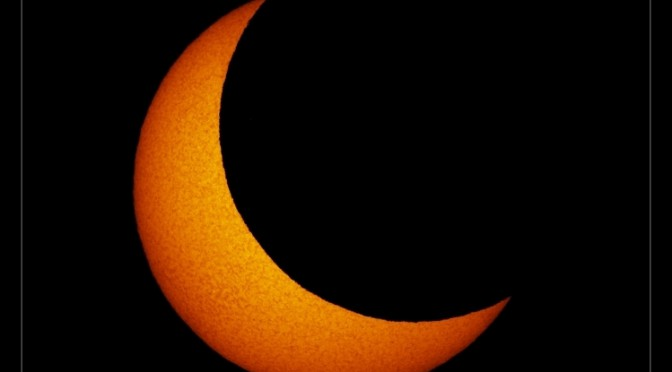# Eclipse Calculations using Python

On 20th March there is a deep partial solar eclipse over the  UK which is total over the Faroe Islands and Svalbard. I set about trying to determine the time of maximum eclipse, and percentage eclipsed at a given location.

I used the pyephem module which is a Python implementation of the numerical routines that are behind the excellent Xephem. The module will allow a user to calculate the positions of astronomical objects (eg Sun, Moon, etc) from a given location at a given time. Using the positions of these objects it’s possible to determine the separation of the centres of these objects.

To work out the percentage eclipse at that time, I used the formula on the Wolfram Mathworld page on “Lunes” (author: Weisstein, Eric W). A “Lune” is the “plane figure bounded by two circular arcs of unequal radii, i.e., a crescent.” which exactly describes the visible surface of the sun during an eclipse.

With the area of the lune calculated, it’s trivial to work out the percent eclipse at that time – and by looping over time, it’s possible to get a list of lists to search for a maximum.